# Marginal Cost Formula

Marginal cost is the expense incurred to produce one additional unit of a good or service

The marginal cost of something is the expense incurred to produce one additional unit of a good or service. It is the change in total cost for an incremental change in production. It is calculated by dividing the change in total cost by the change in output.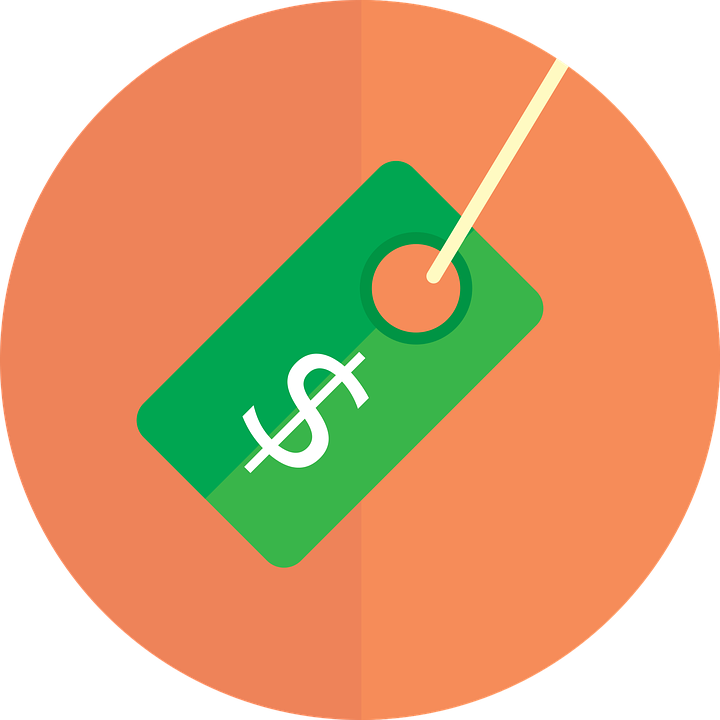Economists often like to "think at the margin," referring to the idea that decisions depend heavily on the margin. This means that people make decisions based on the marginal cost and marginal benefit, meaning the cost and benefit people receive from one more of something.

Theoretically, the point where the marginal cost starts to exceed the marginal benefit is where rational decision-makers cease to produce or consume one more of something.

It is a crucial concept in economics as it describes the drivers of the decision-making process and can help consumers understand prices and suppliers to optimize their production.

Marginal cost is essential for understanding the profit-maximizing output level because it is what you pay for each additional output unit. Therefore, when marginal costs are declining, the company has reduced its average cost per unit because of economies of scale or learning curve benefits.## What Is Marginal Cost

Marginal cost is the cost one incurs from producing an additional unit of goods or services. Typically, the marginal cost increases with each additional unit. On the other hand, the marginal benefit is the utility one gains from one additional unit and decreases with each additional unit.

Real World Example

For example, take the act of eating pizza. While a person may be initially hungry and really enjoy their first slice of pizza, they are likely to enjoy the second slice slightly less than the first. Eventually, the person will no longer be hungry, and the thought of eating more pizza will not be appealing. One might even get sick if one were to overeat pizza.

This principle holds true in production as well as consumption. Hiring an employee may increase production for a business with no employees, but a second employee will likely increase production by a smaller factor. After several employees have been hired, the sales they make will likely be less than the cost of their wages.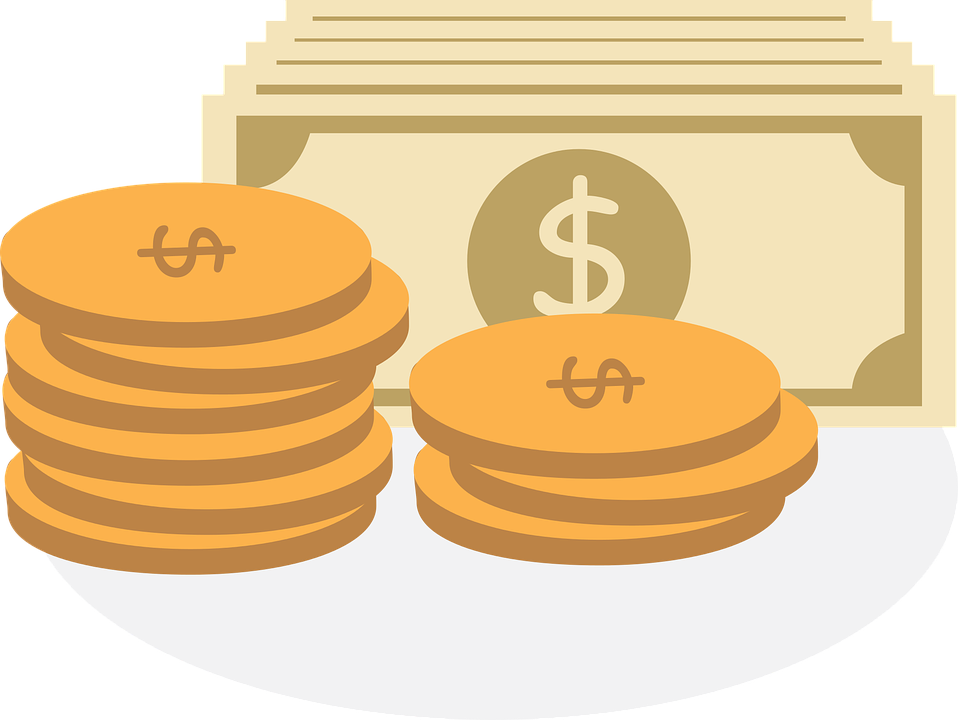This helps businesses decide how much to produce. It is important as it helps understand the profit-maximizing level of output. When marginal costs are declining, it means that the company is reducing its average cost per unit because of economies of scale or learning curve benefits.

## What is the Formula for Marginal Cost

Marginal cost can be calculated as the change in total cost divided by the change in output. The formula is: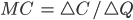where,

• MC = Marginal Cost
• C = Total Cost
• Q = Quantity

The change in total cost is simply the amount spent to produce the extra unit. Similarly, a change in quantity is the number of additional units produced.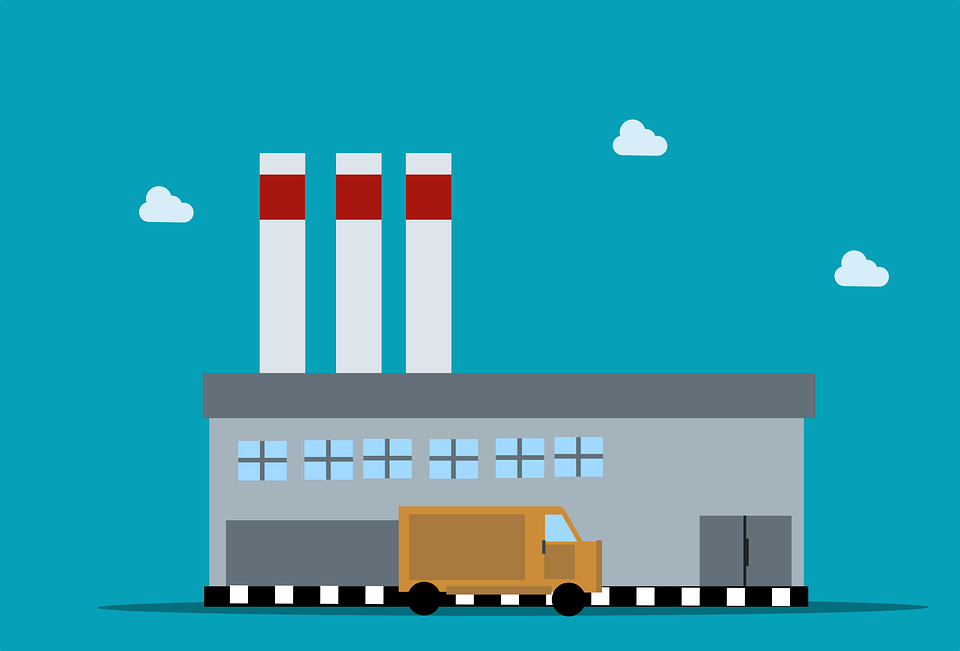Imagine a company decides to increase its production from 10 units to 12 units, and the total cost increases from \$20 to \$26. The change in quantity would be 2 units, and the change in total costs would be \$6.

Dividing change in total cost by the change in quantity is equal to the marginal cost of producing these units. In this example, the marginal cost of each of these units would equal \$3.

In the above example, the marginal cost of producing two additional units was greater than the average total cost. The average total cost of products before the addition of two units was only \$2 per unit (\$20/10), which is lower than the \$3 cost of the additional units. The production of these units increases the average total cost of production to \$2.17 (\$26/12).

An increase in marginal cost is not usually a good sign unless accompanied by a higher increase in marginal selling price.### Everything You Need To Build Your Accounting Skills

To Help you Thrive in the Most Flexible Job in the World.

## Applications Of Marginal Cost

The marginal cost has many applications in business pertaining to the cost of production, especially when deciding how much a company is willing to produce.

Cost is made up of two components: variable costs and fixed costs.

Variable costs are the costs a company incurs that depend on the number of units a company produces. This might include inputs such as labor and materials, as the more a company wishes to produce, the more materials and labor it will take to produce.

Fixed costs are costs that do not change no matter how much a firm produces. This includes costs like rent or some machinery operating expenses.

For example, rent of \$800/mo and machine expense of \$400/mo to operate are fixed costs since they are incurred regardless of how much a firm is able or willing to produce.

Fixed costs, however, are often variable in the long run, such as if a company decided to rent another building and employ more machines to produce more products. As a result, total variable costs typically increase the more units produced, while total fixed costs remain constant with production.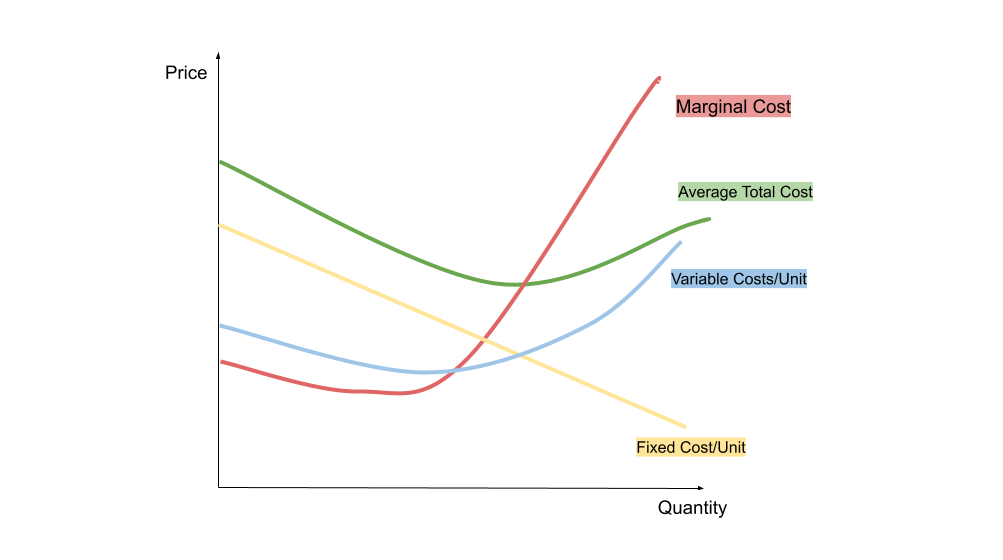Marginal cost, along with fixed costs and variable costs, can tell companies a lot. In a perfectly competitive market, marginal cost is the price level in the market. While real markets are hardly ever perfectly competitive, this concept is still helpful for businesses.

If the marginal cost is below average variable cost in a perfectly competitive market, the firm should shut down. This is because it would lose money (keeping in mind that the price level is the same marginal cost).

On the other hand, if the marginal cost is above average variable costs, but below average total cost, a firm will still lose money. However, it should still stay open in the short run as it will lose less money to operate than shut down. If this were to continue, this firm would likely shut down in the long run.

Where average total cost equals marginal cost, there is both zero profit and zero loss. In a perfectly competitive market, firms will enter and exit the market so that marginal cost is always equal to the average total cost.

As mentioned above, markets are hardly ever perfectly competitive. Firms are most often classified as either oligopolies or competitive monopolies. Competitive monopolies are markets where there are many sellers and buyers, but where their products are slightly different, giving them stronger pricing power.

In this type of market, price is not equal to marginal cost. Instead, these businesses price their products by finding the intersection of marginal cost and marginal revenue, and the price is equal to the demand at that quantity produced.

On the other hand, oligopolies are markets with the same product but with very few sellers. Oligopolies do not use marginal cost to price goods, instead of using something like the Nash equilibrium to find the right price level.While these concepts are clearly based on theoretical economics, they are still instrumental in real-life applications for businesses and investors alike. For example, they can help investors understand better how companies make decisions and improve their analysis skills.

## Economies of Scale

Economies of scale are yet another important application of marginal cost. This is when the average cost of production decreases the more a company produces. The marginal cost must remain below the average total cost for this to happen.

This concept was outlined by Adam Smith, who felt that this could be achieved through labor division. The higher the level of production, the greater the labor can be divided, leading to a maximization of output.Economies of scale are the goal for most companies. Expanding while maintaining or increasing profits is ideal for a business. It allows the company to grow and generate higher profits for the organization.

Economies of scale have the potential to cause what is called a natural monopoly. This is when a company has an advantage over its competitors by entering the market first. This means they can keep the price low and unsustainable for new entrants, leading to a monopoly.

The opposite of economies of scale is called diseconomies of scale. This is when the average cost of production increases the more units are produced. This occurs because marginal cost is above the average total cost. Diseconomies of scale are usually bad for businesses because they cannot expand efficiently. If a company increases production at diseconomies of scale, it risks average total cost becoming greater than its average profits. This would mean the company is losing money and cannot meet demand without going bankrupt.### Everything You Need To Build Your Accounting Skills

To Help you Thrive in the Most Flexible Job in the World.